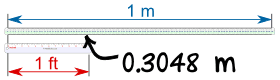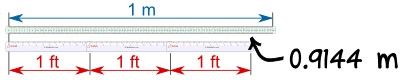# Conversion of Length

Note: we also have Conversion Charts, and a Unit Converter.

## How to Convert Lengths

To convert length from one unit to another: multiply by the correct number.

• Find the correct conversion number (see Conversion Charts)
• Then multiply by that number

Let's look at a detailed example:

### Example: Convert 3 feet into metersWhen we put a 1-meter ruler next to a
1-foot ruler, they look like thisLook closely and we see that the 1-foot ruler comes to exactly 0.3048 on the meter ruler

So, the conversion for feet to meters is: 1 ft = 0.3048 meters

To convert feet to meters, multiply by 0.3048

In fact we could put three 1 foot rulers next to each other like this:Now you can see that 3 feet = 3 × 0.3048 meters = 0.9144 meters

So: 3 ft = 3 × 0.3048 m = 0.9144 m

### Example: Convert 5 kilometers into miles

The conversion for kilometers into miles is:

1 km = 0.6214 mile (to 4 digits of accuracy)

So, the length conversion is "multiply by 0.6214":

5 × 0.6214 = 3.107

So: 5 km = 3.107 miles (to 3 decimal places)

## More or Less?

Sometimes you can get the conversion the "wrong way around", so it is a good idea to think "will my answer be more or less?"

If you get the wrong answer, try dividing by the conversion number.

### Example: You want to convert 8 meters into feet

Will the answer be more or less than 8? Feet are smaller than meters, so there should be more feet than meters.

The conversion is 1 ft = 0.3048 meters.

To the nearest 0.1 feet:

• try multiplying: 8 × 0.3048 = 2.5 feet (oops! that is smaller!)
• try dividing: 8 ÷ 0.3048 = 26.2 feet (that looks good)

Answer: 8 m = 8 ÷ 0.3048 = 26.2 ft